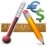# Calculators for Android

Among our compilation of calculators for Android you'll be able to find tools to carry out all sorts of maths operations from your smartphone or tablet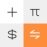Mi Calculator 13.0.13

The calculator of Xiaomi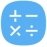Samsung's calculator for Android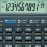The most famous calculators on your phone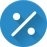Calculate percentages no sweat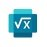Microsoft Math Solver 23.3.401205602

The Microsoft calculator for solving multiple mathematical operations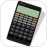A complete scientific calculator on your phone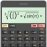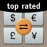A currency converter and a calculator in one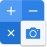Carry out all sorts of calculations on your Android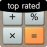Turn your phone into a powerful calculator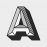Algemator 2.6.6

Solve algebra and trigonometry math problems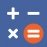ClevCalc 2.19.3

One of the most complete calculators for Android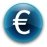Tool to learn about currency conversion